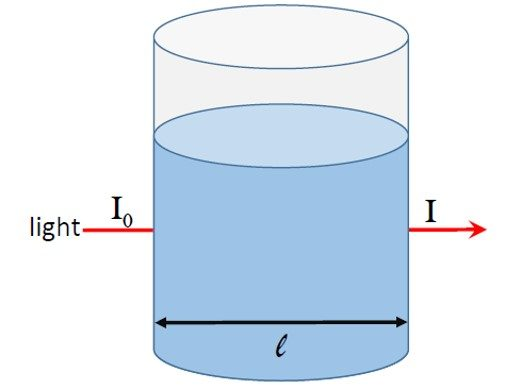# Beer-Lambert Law Calculator

Created by Purnima Singh, PhD
Reviewed by Steven Wooding
Last updated: Apr 06, 2022

Omni's Beer-Lambert law calculator allows you to calculate the absorbance (or attenuation) of light as it passes through any material. You can also use this calculator to determine the molar concentration of solutions. Read on to know what Beer's law is and the formula for Beer's law calculations. You will also find how to calculate concentration from absorbance in Beer's law.

## What is Beer's law (Beer-Lambert law)?

is also known as Beer's law or Beer-Lambert–Bouguer law. It gives a relationship between the concentration of a solution and the attenuation of light as it passes through the solution.

Beer's law states that when a beam of electromagnetic radiation passes through a sample (usually a solution), its absorbance depends on the concentration of the sample and the path length of the beam in the sample.

## Beer's law equation

To understand absorbance, let us consider figure 1. A light beam of intensity  Io passes through a solution placed in a container of diameter l.Figure 1: Absorption of light as it passes through a solution.

If the solution absorbs light, the intensity of the light emerging from the container will be less than  Io. If the intensity of the transmitted light is I, we can define the absorbance A as:

 A = log10(Io / I)

We can express the Beer's law equation as:

 A = log10(Io / I) = ε * l * c

where:

• ε — Molar absorption coefficient or the molar absorptivity;
• l — Path length of the beam in the sample; and
• c — Concentration of the solution.

In spectroscopy, the path length is usually expressed in cm and absorbance is unitless (a dimensionless quantity). The unit for expressing the concentration of sample solution is mol/L, and hence the units of molar absorptivity are L/mol•cm.

## How to use the Beer-Lambert law calculator

Let us see how to use the Beer's law calculator to calculate the absorbance of light by a solution of molar concentration 4.33 * 10-5 mol.L-1. Let the path length be 1 cm and the molar absorptivity be 8400 M-1cm-1.

1. Enter the value of the molar absorption coefficient, i.e., 8400 M-1cm-1.
2. Type the concentration of the solution, i.e., 43.3 µmol.L-1.
3. Input the path length, i.e., 1 cm.
4. The calculator will display the absorbance: 0.3637.
5. You can also use this Beer-Lambert law calculator as a transmittance to absorbance calculator. Just enter the transmittance values in percentage, and you will get the absorbance.

## Absorbance to transmittance conversion

In figure 1, the fraction of light that passes through the sample gives the transmittance T, i.e.:

T = I / Io

…and we can express the relation between transmittance and absorbance as:

A = log10(1 / T)

As we note, the relation between absorbance and transmittance is logarithmic. An absorbance of 0 implies a transmittance of 100%.

In spectrophotometry techniques, we measure the intensity of the radiation entering the sample solution and the intensity of radiation coming out of it. We then use the two intensities to calculate the transmittance or absorbance values.

## Applications of the Beer-Lambert law

Most of the spectroscopic analysis techniques in chemistry are based on the Beer-Lambert law. Some common applications of Beer's law in analytical chemistry are:

• To determine the concentration of samples by measuring the absorbance.
• To determine the identity of an unknown substance by determining its molar absorptivity.

## FAQ

### What is the unit of absorbance in Beer's law?

The absorbance is a unitless quantity. It is the ratio of the intensity of the incident light and the transmitted light; hence, it is dimensionless and has no units. However, sometimes absorbance is reported in absorbance units (AU).

### How do I calculate molar absorptivity from Beer's law?

To calculate molar absorptivity from Beer's law, proceed as follows:

1. Multiply the path length with the molar concentration of the solution.
2. Divide the absorbance by the value obtained in step 1.
3. Congrats! You have successfully calculated the molar absorptivity from Beer's law.

### How do I calculate concentration from absorbance in Beer's law?

Beer-Lambert law is often used in determining the concentration of solutions. To calculate the concentration of a solution from Beer's law, follow the given instructions:

1. Determine the absorbance as the light of a given wavelength passes through the solution.
2. Find out the path length the light has to travel.
3. Multiply the molar absorption coefficient with the path length.
4. Divide the absorbance by the value obtained in step 3, and you will get the concentration of the solution.

### How do I calculate transmittance from absorbance?

To calculate transmittance from absorbance, we need to follow the given steps:

1. Subtract the absorbance value from the number 2.
2. Take the antilog of the value obtained in step 1, and you will get the transmittance percentage.
3. You can also use our Beer-Lambert law calculator to calculate transmittance.
Purnima Singh, PhD
Molar absorption coefficient
/
M
•cm
Concentration
mol
/L
Path length
cm
Absorbance
Transmittance
%
People also viewed…

### Lost socks

Socks Loss Index estimates the chance of losing a sock in the laundry.

### Nernst equation

The Nernst equation calculator applies the fundamental electrochemistry equation to find the reduction potential in a cell reaction.

### Percentage concentration to molarity

With our tool, you can easily convert percentage concentration to molarity.

### Sunbathing

Do you always remember to put on sunscreen before going outside? Are you sure that you use enough? The Sunbathing Calculator ☀ will tell you when's the time to go back under an umbrella not to suffer from a sunburn!